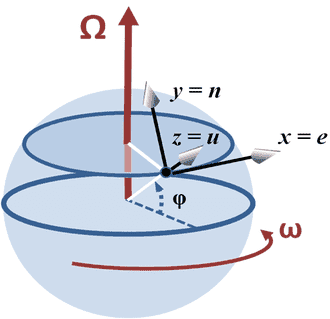# Train experiencing Coriolis force

## Homework Statement

A train at latitude λ in the northern hemisphere is moving due north with a speed v along
a straight and level track. Which rail experiences the larger vertical force? Show that the ratio
R of the vertical forces on the rails is given approximately by:

R= 1+ 8Ωvh sin λ / ga

where h is the height of the centre of mass of the train above the rails which are a distance a
apart, g is the acceleration of free fall, and Ω is the angular velocity of the Earth’s rotation.

## Homework Equations

1. Coriolis force: 2mΩv sin λ
2. Weight of train, mg.

## The Attempt at a Solution

I have figured out that the eastward track should experience a larger force, as the Earth is rotating from west to east and the train will tend to veer towards the east. I understand that the Coriolis force vector points to the right on the Northern Hemisphere.

For the second part of the question, I considered the forces acting on the tracks. The tracks should experience a vertical force due to the weight of the train, and a horizontal force due to the Coriolis force. However if the Coriolis force acts horizontally, how does it contribute to the vertical force?

Or should I consider the 3D rotation vector with a coordinate system that corresponds to (x,y,z)=(north, east, upwards) as shown by this diagram? (from http://en.wikipedia.org/wiki/Coriolis_effect)I thought that if I considered only the component along the z-axis to obtain the vertical force. But how would it relate to the spacing, a, between the tracks?

BvU
Homework Helper
Hello xy, welcome to PF :)

Where does the Coriolis force act ?
You are given some quantitative answer that contains a and h. Must be a clue in there !

I suppose that the ratio h/a hints that the problem involves tan. Here's my attempt:

Vertical component of horizontal Coriolis force = 2 mΩvsinλ tan θ = 4 mΩvsinλ h /a (since tan θ = 2h/a)

Assuming that weight, mg is distributed evenly across both rails, weight on each wheel is mg/2.

Hence one rail experiences vertical force= weight plus Coriolis force: 4 mΩvsinλ h /a + mg/2

The other rail experiences vertical force mg/2

The ratio of the forces is R= 1+ 8 Ωvsinλ h / ga as required by question.

I hope that my understanding is correct!

haruspex
Homework Helper
Gold Member
if the Coriolis force acts horizontally, how does it contribute to the vertical force?
Excellent question. BvU asked where the Coriolis force acts on the train. Draw a free body diagram of the train, seen end-on. What, despite the Coriolis force, keeps the train heading in the right direction? Where does that force act?

BvU
Homework Helper
Hehe, usually the vertical component of a horizontal force is zero, so this line of reasoning is very questionable :) .
That's what you get rather easily when the answer is available already.

Perhaps I wrong-footed you with my hinting question. If you draw a little diagram, you'll see that mg is offset by two normal forces from the rails that add up to mg.

Fc is offset by a horizontal component exercised by the eastern rail (and/or friction in the east-west directtion from both rails). Those two constitute a torque that also has to be offset, namely by NOT distributing mg evenly over both rails!

•Chestermiller
Working on the same problem at the moment, invoked Newtons second law in the vertical direction, mg = sum of reaction forces, then used moments about one of the wheels, and substituted to remove 'm' then found the ratio of the reaction forces of the right wheel to the left which I think is the correct approach. However the answer i get differs from what's required, not sure why.

haruspex
Homework Helper
Gold Member
Working on the same problem at the moment, invoked Newtons second law in the vertical direction, mg = sum of reaction forces, then used moments about one of the wheels, and substituted to remove 'm' then found the ratio of the reaction forces of the right wheel to the left which I think is the correct approach. However the answer i get differs from what's required, not sure why.
That's all fascinating, but we cannot locate your error if you do not post your working.

My bad, here's the equations I've used that lead to the wrong result:

Newtons II law: mg=R1+R2

mag/2 + 2mΩvhsin λ - aR2 =0

Where R1 and R2 are the reaction forces on the left and right wheels respectively and the Coriolis force acts horizontally in the East direction.

Then I rearranged to find R2/R1, eliminating the mass from both eqs.

haruspex
Homework Helper
Gold Member
Then I rearranged to find R2/R1, eliminating the mass from both eqs.
... and it is somewhere in there that you are not making the right step.
At a guess, you are not taking into account the word "approximately" in the question.

•QuanTom1
... and it is somewhere in there that you are not making the right step.
At a guess, you are not taking into account the word "approximately" in the question.
You were right, just needed a first order Taylor expansion at the end, cheers!